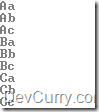# Rewrite Nested ForEach Loop in LINQ

 Tweet

Let’s assume you have two string array's of the same length and you need to loop through each element of the array and concatenate it’s elements. One way to do this is to use nested foreach loops as shown below

`string[] uCase = { "A", "B", "C" };string[] lCase = { "a", "b", "c" };foreach (string s1 in uCase){    foreach (string s2 in lCase)    {        Console.WriteLine(s1 + s2);    }}`

Now if you want to get the same results in LINQ without using a nested foreach, use the IEnumerable.SelectMany which projects each element of a sequence and flattens the resulting sequences into one sequence

`string[] uCase = { "A", "B", "C" };string[] lCase = { "a", "b", "c" };var ul = uCase.SelectMany(    uc => lCase, (uc, lc) => (uc + lc));foreach (string s in ul){    Console.WriteLine(s);}`

OUTPUT